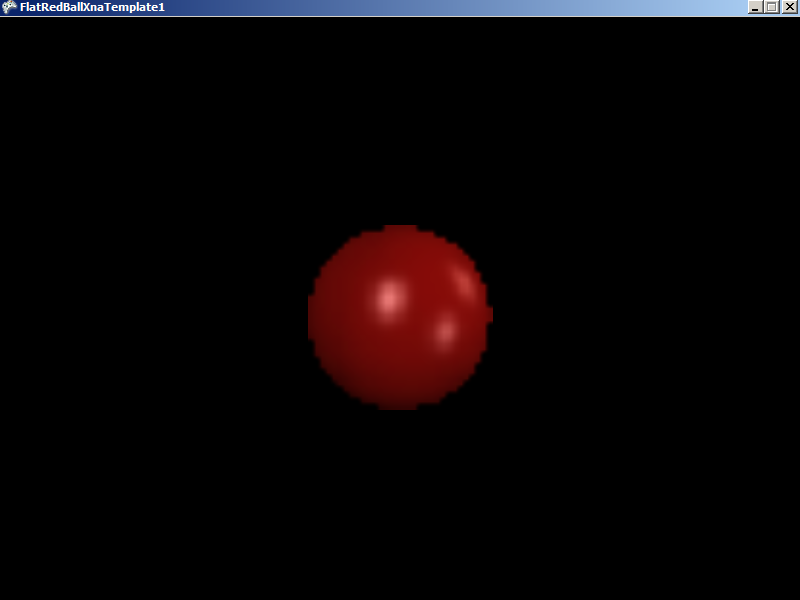# OrthogonalHeight

## Introduction

The OrthogonalHeight and OrthogonalWidth values control how many units tall and wide the view of the Camera can be seen. These values apply if the camera is 2D (if the Orthogonal value is set to true). If using Glue, the default setup is for a 2D camera.

Orthogonal cameras are useful for creating 2D games, or for creating menu systems which work in pixels.

## Visualizing Orthogonal Values

By default FlatRedBall games use a 2D camera with an OrthogonalWidth of 800 and OrthogonalHeight of 600. The following diagram can help visualize this configuration:## Code Example – Using Resolution to Position Objects at the Edge of the Screen

The OrthogonalHeight and OrthogonalWidth values can be used to position an object at the edge of the screen. Keep in mind that a Camera’s X and Y values represent the center of the screen, so the edges of the screen can be obtained by adding or subtracting half of the OrthogonalWidth or OrthogonalHeight.

```float leftEdge = Camera.Main.X - Camera.Main.OrthogonalWidth/2.0f;
float rightEdge = Camera.Main.X + Camera.Main.OrthogonalWidth/2.0f;
float topEdge = Camera.Main.Y + Camera.Main.OrthogonalHeight/2.0f;
float bottomEdge = Camera.Main.Y - Camera.Main.OrthogonalHeight/2.0f;```

## Setting the Camera to 2D

The Camera’s UsePixelCoordinates method sets the calling Camera’s Orthogonal property to true and adjusts the pixel coordinates to the screen’s resolution. In other words, if the screen is 800X600, calling UsePixelCoordinates sets the OrthogonalWidth to 800 and the OrthogonalHeight to 600.

## Orthogonal Width/Orthogonal Height As Windows into the World

OrthogonalWidth and OrthogonalHeight control how much of the world can be seen. For example, consider the following game level which is 3200×3200 pixels (zoomed down to fit on screen):If this level were viewed with a camera with OrthogonalWidth of 800 and an OrthogonalHeight of 480, the red square represents the area that might be visible at one time:The following image shows what this might look like in a FlatRedBall game:## Zooming using Orthogonal values

When using a 3D camera (Orthogonal = false) the most common way to perform a “zoom in” is to adjust the Camera’s Z value. When objects move closer to the Camera in a perspective view, they become larger. This is not the case when the Camera is Orthogonal.

The following code adjusts the Camera’s OrthogonalHeight and OrthogonalWidth (through the FixAspectRatioYConstant method) by using the up/down keys on the keyboard.

Add the following to Initialize after initializing FlatRedBall:

```SpriteManager.Camera.UsePixelCoordinates(false);

Sprite sprite = SpriteManager.AddSprite("redball.bmp");
sprite.PixelSize = .5f;
```

Add the following to Update:

```if (InputManager.Keyboard.KeyDown(Keys.Down))
{
SpriteManager.Camera.OrthogonalHeight += 200 * TimeManager.SecondDifference;
SpriteManager.Camera.FixAspectRatioYConstant();
}
if (InputManager.Keyboard.KeyDown(Keys.Up))
{
SpriteManager.Camera.OrthogonalHeight -= 200 * TimeManager.SecondDifference;
SpriteManager.Camera.FixAspectRatioYConstant();
}
```